Share

Books Shortlist

# NCERT solutions for Class 10 Mathematics chapter 12 - Areas Related to Circles

## Mathematics Textbook for Class 10

#### NCERT Mathematics Class 10## Chapter 12: Areas Related to Circles

Ex. 12.10Ex. 12.20Ex. 12.30

#### Chapter 12: Areas Related to Circles Exercise 12.10 solutions [Pages 225 - 226]

Ex. 12.10 | Q 1 | Page 225

The radii of two circles are 19 cm and 9 cm respectively. Find the radius of the circle which has circumference equal to the sum of the circumferences of the two circles.

Ex. 12.10 | Q 2 | Page 225

The radii of two circles are 8 cm and 6 cm respectively. Find the radius of the circle having area equal to the sum of the areas of the two circles.

Ex. 12.10 | Q 3 | Page 225

Given figure depicts an archery target marked with its five scoring areas from the centre outwards as Gold, Red, Blue, Black and White. The diameter of the region representing Gold score is 21 cm and each of the other bands is 10.5 cm wide. Find the area of each of the five scoring regions. [use π = 22/7]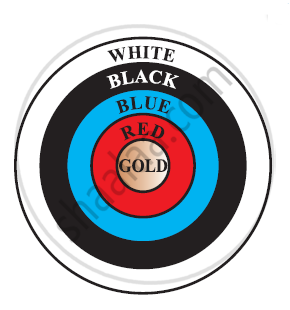Ex. 12.10 | Q 4 | Page 226

The wheels of a car are of diameter 80 cm each. How many complete revolutions does each wheel make in 10 minutes when the car is traveling at a speed of 66 km per hour? [Use Π = 22/7]

Ex. 12.10 | Q 5 | Page 226

Tick the correct answer in the following and justify your choice: If the perimeter and the area of a circle are numerically equal, then the radius of the circle is

• 2 units

• π units

• 4 units

• 7 units

#### Chapter 12: Areas Related to Circles Exercise 12.20 solutions [Pages 230 - 231]

Ex. 12.20 | Q 1 | Page 230

Find the area of a sector of a circle with radius 6 cm if angle of the sector is 60° [Use Π = 22/7]

Ex. 12.20 | Q 2 | Page 230

Find the area of a quadrant of a circle whose circumference is 22 cm. [Use pi = 22/7]

Ex. 12.20 | Q 3 | Page 230

The length of the minute hand of a clock is 14 cm. Find the area swept by the minute hand in 5 minutes.  [Use Π = 22/7]

Ex. 12.20 | Q 4 | Page 230

A chord of a circle of radius 10 cm subtends a right angle at the centre. Find the area of the corresponding:

(i) Minor segment

(ii) Major sector

[Use π = 3.14]

Ex. 12.20 | Q 5 | Page 230

In a circle of radius 21 cm, an arc subtends an angle of 60° at the centre. Find:

(i) The length of the arc

(ii) Area of the sector formed by the arc

(iii) Area of the segment forced by the corresponding chord

[use Π = 22/7]

Ex. 12.20 | Q 6 | Page 230

A chord of a circle of radius 15 cm subtends an angle of 60° at the centre. Find the areas of the corresponding minor and major segments of the circle. [Use π = 3.14 and sqrt3 = 1.73]

Ex. 12.20 | Q 7 | Page 230

A chord of a circle of radius 12 cm subtends an angle of 120° at the centre. Find the area of the corresponding segment of the circle. [Use π = 3.14 and sqrt3 = 1.73 ]

Ex. 12.20 | Q 8 | Page 230

A horse is tied to a peg at one corner of a square shaped grass field of side 15 m by means of a 5 m long rope (see the given figure). Find

(i) The area of that part of the field in which the horse can graze.

(ii) The increase in the grazing area of the rope were 10 m long instead of 5 m.

[Use π = 3.14]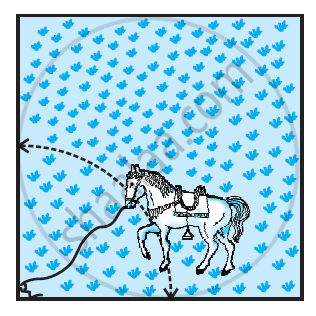Ex. 12.20 | Q 9 | Page 230

A brooch is made with silver wire in the form of a circle with diameter 35 mm. The wire is also used in making 5 diameters which divide the circle into 10 equal sectors as shown in figure. Find.

(i) The total length of the silver wire required.

(ii) The area of each sector of the brooch

[Use Π = 22/7]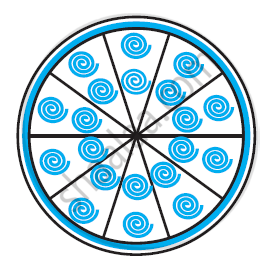Ex. 12.20 | Q 10 | Page 231

An umbrella has 8 ribs which are equally spaced. Assuming umbrella to be a flat circle of radius 45 cm. Find the area between the two consecutive ribs of the umbrella.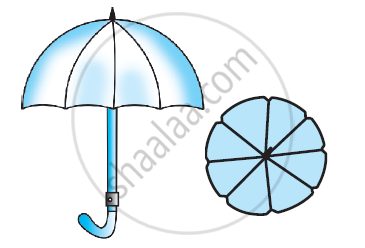Ex. 12.20 | Q 11 | Page 231

A car has two wipers which do not overlap. Each wiper has blade of length 25 cm sweeping through an angle of 115°. Find the total area cleaned at each sweep of the blades [Use Π  = 22/7]

Ex. 12.20 | Q 12 | Page 231

To warn ships for underwater rocks, a lighthouse spreads a red coloured light over a sector of angle 80° to a distance of 16.5 km. Find the area of the sea over which the ships warned. [Use π = 3.14]

Ex. 12.20 | Q 13 | Page 231

A round table cover has six equal designs as shown in figure. If the radius of the cover is 28 cm, find the cost of making the designs at the rate of Rs.0.35 per cm2. [Use sqrt3 = 1.7]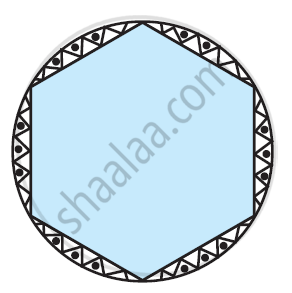Ex. 12.20 | Q 14 | Page 231

Tick the correct answer in the following:

Area of a sector of angle p (in degrees) of a circle with radius R is

• p/180  x 2ΠR

• p/180 x Π R2

• p/360 x 2Π R

• p/720 x 2Π R2

#### Chapter 12: Areas Related to Circles Exercise 12.30 solutions [Pages 234 - 238]

Ex. 12.30 | Q 1 | Page 234

Find the area of the shaded region in the given figure, if PQ = 24 cm, PR = 7 cm and O is the centre of the circle. [Use Π = 22/7]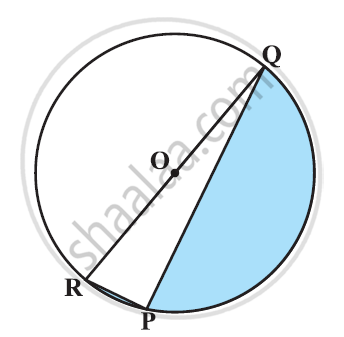Ex. 12.30 | Q 2 | Page 235

Find the area of the shaded region in the given figure, if radii of the two concentric circles with centre O are 7 cm and 14 cm respectively and ∠AOC = 40° [Use Π = 22/7]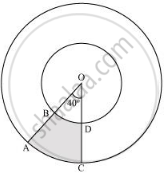Ex. 12.30 | Q 3 | Page 235

Find the area of the shaded region in the given figure, if ABCD is a square of side 14 cm and APD and BPC are semicircles.  [Use Π = 22/7]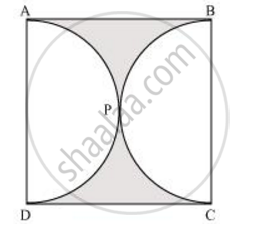Ex. 12.30 | Q 4 | Page 235

Find the area of the shaded region in the given figure, where a circular arc of radius 6 cm has been drawn with vertex O of an equilateral triangle OAB of side 12 cm as centre [Use Π = 22/7]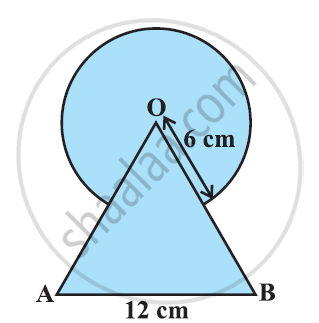Ex. 12.30 | Q 5 | Page 235

From each corner of a square of side 4 cm a quadrant of a circle of radius 1 cm is cut and also a circle of diameter 2 cm is cut as shown in the given figure. Find the area of the remaining portion of the square [Use Π = 22/7]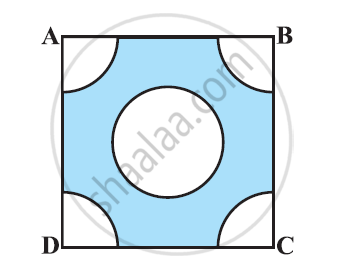Ex. 12.30 | Q 6 | Page 235

In a circular table cover of radius 32 cm, a design is formed leaving an equilateral triangle ABC in the middle as shown in the given figure. Find the area of the design (Shaded region). [Use Π = 22/7]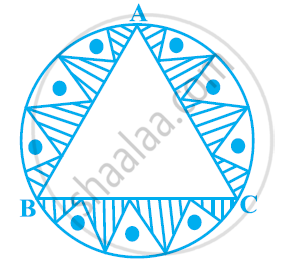Ex. 12.30 | Q 7 | Page 236

In the given figure, ABCD is a square of side 14 cm. With centres A, B, C and D, four circles are drawn such that each circle touches externally two of the remaining three circles. Find the area of the shaded region.  [Use Π = 22/7]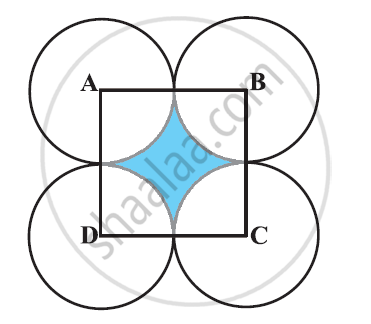Ex. 12.30 | Q 8 | Page 236

The given figure depicts a racing track whose left and right ends are semicircular.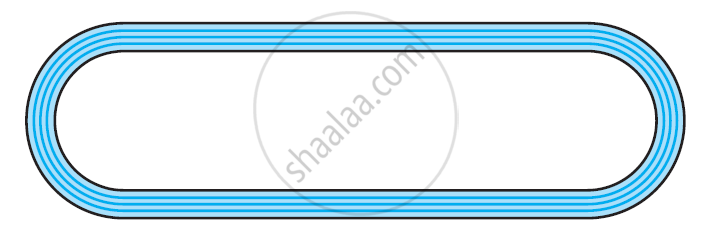The distance between the two inner parallel line segments is 60 m and they are each 106 m long. If the track is 10 m wide, find:

(i) The distance around the track along its inner edge

(ii) The area of the track

[Use Π = 22/7]

Ex. 12.30 | Q 9 | Page 236

In the given figure, AB and CD are two diameters of a circle (with centre O) perpendicular to each other and OD is the diameter of the smaller circle. If OA = 7 cm, find the area of the shaded region.  [Use Π = 22/7]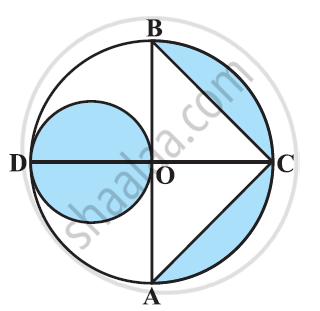Ex. 12.30 | Q 10 | Page 236

The area of an equilateral triangle ABC is 17320.5 cm2. With each vertex of the triangle as centre, a circle is drawn with radius equal to half the length of the side of the triangle (See the given figure). Find the area of shaded region. [Use π = 3.14 and sqrt3 = 1.73205]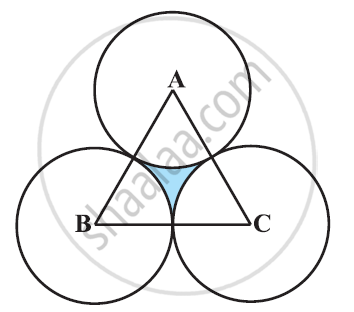Ex. 12.30 | Q 11 | Page 237

On a square handkerchief, nine circular designs each of radius 7 cm are made (see the given figure). Find the area of the remaining portion of the handkerchief.[Use Π = 22/7]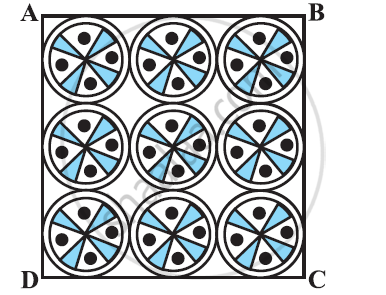Ex. 12.30 | Q 12 | Page 237

In the given figure, OACB is a quadrant of circle with centre O and radius 3.5 cm. If OD = 2 cm, find the area of the

[Use Π = 22/7]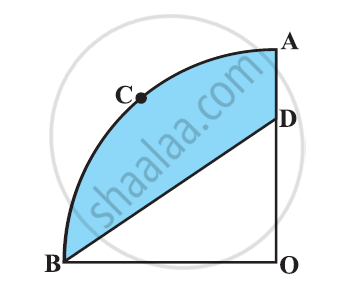Ex. 12.30 | Q 13 | Page 237

In fig. 3, a square OABC is inscribed in a quadrant OPBQ of a circle. If OA = 20 cm, find the area of the shaded region. (Use π = 3.14)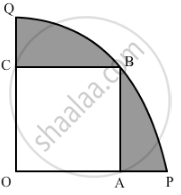Ex. 12.30 | Q 14 | Page 237

AB and CD are respectively arcs of two concentric circles of radii 21 cm and 7 cm and centre O (see the given figure). If ∠AOB = 30°, find the area of the shaded region. [Use Π = 22/7]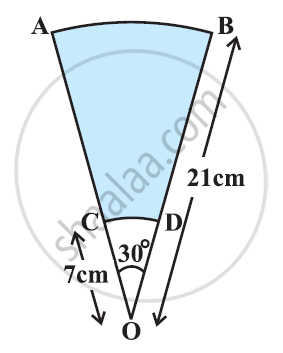Ex. 12.30 | Q 15 | Page 237

In the given figure, ABC is a quadrant of a circle of radius 14 cm and a semicircle is drawn with BC as diameter. Find the area of the shaded region. [Use Π = 22/7]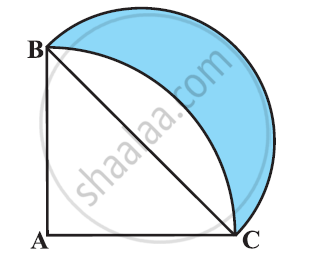Ex. 12.30 | Q 16 | Page 238

Calculate the area of the designed region in the given figure common between the two quadrants of circles of radius 8 cm each. [Use Π = 22/7]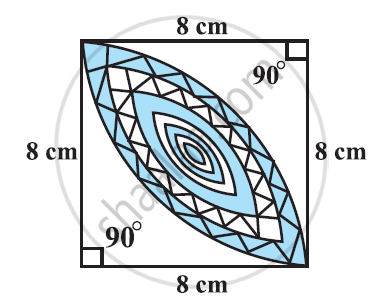## Chapter 12: Areas Related to Circles

Ex. 12.10Ex. 12.20Ex. 12.30

#### NCERT Mathematics Class 10## NCERT solutions for Class 10 Mathematics chapter 12 - Areas Related to Circles

NCERT solutions for Class 10 Maths chapter 12 (Areas Related to Circles) include all questions with solution and detail explanation. This will clear students doubts about any question and improve application skills while preparing for board exams. The detailed, step-by-step solutions will help you understand the concepts better and clear your confusions, if any. Shaalaa.com has the CBSE Mathematics Textbook for Class 10 solutions in a manner that help students grasp basic concepts better and faster.

Further, we at shaalaa.com are providing such solutions so that students can prepare for written exams. NCERT textbook solutions can be a core help for self-study and acts as a perfect self-help guidance for students.

Concepts covered in Class 10 Mathematics chapter 12 Areas Related to Circles are Areas of Combinations of Plane Figures, Areas of Sector and Segment of a Circle, Areas Related to Circles Examples and Solutions, Problems Based on Areas and Perimeter Or Circumference of Circle, Sector and Segment of a Circle, Perimeter and Area of a Circle.

Using NCERT Class 10 solutions Areas Related to Circles exercise by students are an easy way to prepare for the exams, as they involve solutions arranged chapter-wise also page wise. The questions involved in NCERT Solutions are important questions that can be asked in the final exam. Maximum students of CBSE Class 10 prefer NCERT Textbook Solutions to score more in exam.

Get the free view of chapter 12 Areas Related to Circles Class 10 extra questions for Maths and can use shaalaa.com to keep it handy for your exam preparation

S